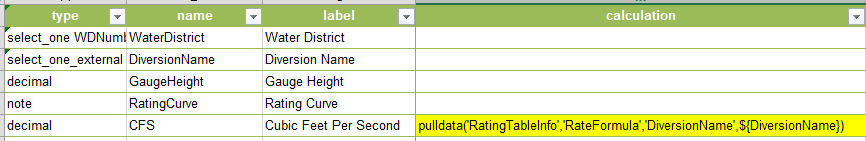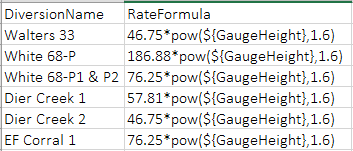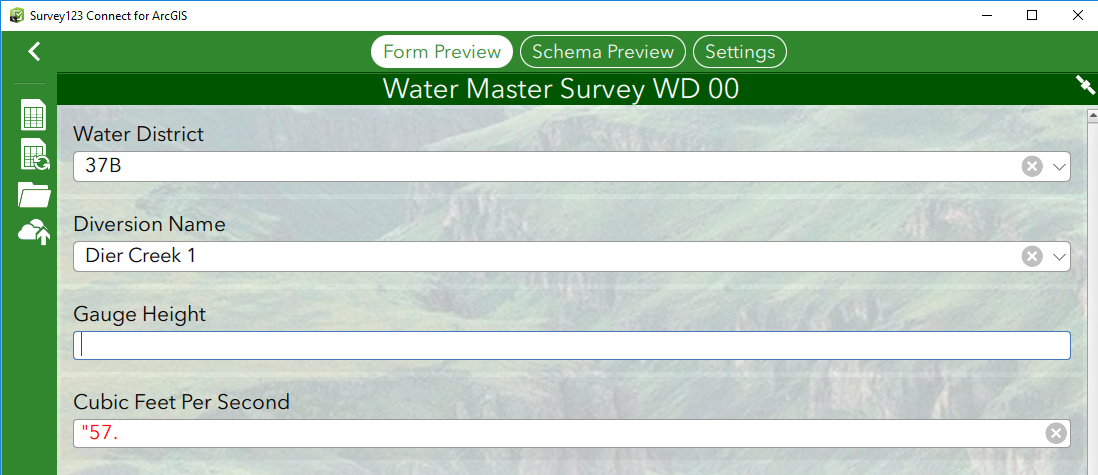# In Calculation Field: Use a pulldata function to get the equation

70
2
01-24-2019 06:29 AM
Highlighted

I am building a survey to record flow measurements for surface water. A user will read a staff gauge in a stream. This reading will then be entered into an equation specific to that stream to convert the reading into a flow expressed in cubic feet per second. I was hoping I could set this up as follows (but it doesn't work, but hopefully illustrates what I am trying to do):The specific formula in the calculation field is thus pulled from a csv table called "RatingTableInfo" which looks as follows:The results, when set up as shown above looks like this:In other words, it just shows the first two numbers of the equation, rather than the result of the equation.  Is there a way to set this up so it actually does the math?

Any suggestion is appreciated.  My alternative thought is to build extensive lookup tables where for each equation I provide all the possible results of the equation, rather than the equation itself and then use the pulldata function against those tables. However, there are dozens of equations and that seems quite a bit more cumbersome.

Thank you!!!

2 Replies
Highlighted
byEsri Regular Contributor

Hi Wilma,

Can you please provide your XLSForm along with the external CSV that you are using for the pulldata calculation?  I'd like to test something on my end.  You can alternatively send it to barmstrong at esri.com.

Thanks,

Brandon

Highlighted
byEsri Regular Contributor

Hi Wilma,

After taking a look at the XLSForm and the CSV you provided, I think your best approach would be separate the calculation out from external CSV.  The idea would be to pull the unique rate formula from the CSV first, and then in a sequential question, reference it in a calculation that includes the formula resulting in Cubic Feet Per Second.

 type name label appearance default calculation choice_filter select_one WDNumber WaterDistrict Water District autocomplete 37B select_one_external DiversionName DiversionName Diversion Name autocomplete WaterDistrictNumber=\${WaterDistrict} decimal GaugeHeight Gauge Height calculate calc calculation for pulldata pulldata('RatingTableInfo','RateFormula','DiversionName',\${DiversionName}) decimal CFS Cubic Feet Per Second (\${calc}*pow(\${GaugeHeight},1.6))I have sent a revised version of the XLSForm and CSV in a reply to your email.

Best,

Brandon